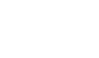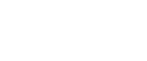## Questions1. The table below shows the masses to the nearest kg of a number of people.
 Mass (kg)Frequency 50 – 5419 55 – 5923 60 – 6440 65 – 6928 70 – 7417 75 – 799 80 – 844
1. Using an assumed mean of 67.0, calculate to one decimal place the mean mass.
2. Calculate to one decimal place the standard deviation of the distribution.
2. Use only a ruler and pair of compasses in this question;
1. construct triangle ABC in which AB = 7cm, BC = 6cm and AC = 5cm
2. On the same diagram construct the circumcircle of triangle ABC and measure its radius
3. Construct the tangent to the circle at C and the internal bisector of angle BAC. If these lines meet at D, measure the length of AD
3. Below is a histogram drawn by a student of Got Osimbo Girls Secondary School.
1. Develop a frequency distribution table from the histogram above.
2. Use the frequency distribution table above to calculate;
1. The inter-quartile range.
2. The sixth decile.
4. ABC is a triangle drawn to scale. A point x moves inside the triangle such that
1. AX 4 cm
2. BX >CX
3. Angle BCX Angle XCA.
Show the locus of X.
5. The following able shows the distribution of marks of 80 students
 Marks 1-10 11-20 21-30 31-40 41-50 51-60 61-70 71-80 81-90 91-100 Frequency 1 6 10 20 15 5 14 5 3 1
1. Calculate the mean mark
2. Calculate the semi-interquartile range
3. Workout the standard deviation for the distribution
6. The table below shows the marks of 90 students in a mathematical test
 Marks 5-9 10-14 15-19 20-24 25-29 30-34 35-39 No. of students 2 13 31 23 14 X 1
1. Find X
2. State the modal class
3. Using a working mean of 22, calculate the;
1. Mean mark
2. Standard deviation
7.
1. Using a ruler and a pair of compasses only construct triangle PQR in which PQ = 5cm, PR = 4cm and ∠PQR = 30o
2. Measure
1. RQ
2. ∠PQR
3. Construct a circle, centre O such that the circle passes through vertices P, Q, and R
4. Calculate the area of the circle
8. The ages of 100 people who attended a wedding were recorded in the distribution table below
 Age 0-19 20-39 40-59 60-79 80-99 Frequency 7 21 38 27 7
1. Draw the cumulative frequency
2. From the curve determine:
1. Median
2. Inter quartile range
3. 7th Decile
4. 60th Percentile
9. The marks obtained by 10 students in a maths test were:-
25, 24, 22, 23, x, 26, 21, 23, 22 and 27
The sum of the squares of the marks, Σx2 = 5154
1. Calculate the
1. value of x
2. Standard deviation
2. If each mark is increased by 3, write down the:-
1. New mean
2. New standard deviation
10. 40 form four students sat for a mathematics test and their marks were distributed as follows:-
 Marks 1 – 10 11-20 21- 30 31 – 40 41 – 50 51 – 60 61 – 70 71 – 80 81 – 90 91 - 100 No. ofstudents 1 3 4 7 12 9 2 1 0 1
1. Using 45.6 as the working mean, calculate;
1. The actual mean.
2. The standard deviation.
2. When ranked from first to last, what mark was scored by the 30th student? (Give your answer correct to 3 s.f.)
11. The table below shows the distribution of marks scored by pupils in a maths test at Nyabisawa Girls.
 Marks 11 – 20 21 – 30 31 – 40 41 – 50 51 – 60 61 – 70 71 – 80 81 – 90 Frequency 2 5 6 10 14 11 9 3
1. Using an Assumed mean 45.5, calculate the mean score.
2. Calculate the median mark.
3. Calculate the standard deviation.
4. State the modal class.
12. The table below shows the marks scored in a mathematics test by a form four class;
 Marks 20-29 30-39 40-49 50-59 60-69 70-79 80-89 No. of students 4 26 72 53 25 9 11
1. Using an assumed mean of 54.5, calculate:-
1. The mean
2. The standard deviation
2. Calculate the inter quartile range1.
 Masskg Midtermx F d=xA fd d2 fd2 50 - 5455 - 5960 - 6465 - 6970 - 7475 - 7980 - 84 52576267727782 19234028179 4 -15-10-50 51015 -285-230-2000859060 22510025025100225 4275230010000425900900 Σf =140 Σfs= -480 Σfd2 = 9800
Marks awarded for table as follows:-
Σf = 140 B1
Column for d B1
Column for fd B1
Σfd = - 480 B1
Column for d2 = 9800 B1
Σfd = 9800B1
x =A + Σfd
Σf
= 67.0 + - 480
140
= 67.0 – 3.43 = 63.57 ………… M1
= 63.6 kg …………… A1
Standard deviation = Σfd2 - Σfd
Σf       Σf2. = 8/150 + 6/150 + 9/300+ 3/300
=40/300 = 2/15
1. Construction of AB B1
Construction of BC B1
Construction of AC B1
2. Construction of bisect of AC B1
Construction of bisect BC B1
3. Construction of bisect ∠ CAB B1 OC B1
3.
1.
 Class f x d = A – x fd d2 fd2 41 – 5051 – 5556 – 6566 – 7071 – 85 2060605015 45.55360.56873 157.50 -7.5-12.5 3004500 -375187.5 22556.25056.25156.25 4500337502812.502343.75 Σfd = 562.5 Σfd2 13031.25
2.4. 15 (ax)4 (-2/x2)2 = 4860
60a4 = 4860
a4= 81
a = 3
5.
 Marks(x) Freq.(f) fx d=x-x d2 Fd2 5.515.525.535.545.555.565.675.585.595.5 1 61020155145 3 1 5.599255710682.5277.5917377.5256.595.5 -40.45-30.45-20.45-10.45-0.459.5519.5529.5539.5549.55 1636927.2418.2109.20.202591.20382.2873.215642455 16365563418221843038456535436646922455 Σf = 80 Σfx = 3676 Σfx2= 33,923
1. Mean = Σfx = 3676
Σf      80
= 45.95
2. Q1 = 30.5 + x 10
14
= 62.64
S.I.R = ½ (62.64 -32)
= 15.32
3. Standard deviation6.
1. x = 90 – (2 +13 + 51 + 27 + 14 + 1)
= 90 – 84 = 6
2. 15 – 19
3.
1.
 Class x f D= x-A fd D2 Fd2 5-9 7 2 -15 -30 225 450 10-14 12 13 -10 -130 100 1300 15-19 17 31 -5 -155 25 775 20-24 22 23 0 0 0 0 25-29 27 14 5 70 350 4900 30-34 32 6 10 60 600 3600 35-39 37 1 15 15 225 225
Ef = 90 Efd = 170 Efd2 = 11250
Mean = E + d + A
Ef
= -170 + 22
90
= 22 – 1.888 = 20.11
2. S.d = Efd - [Efd]2
Ef       Ef
= 122 – (-1.888)2
= 125 – 3.566 = 121.4
= 11.02
7.
1.2.
1. RQ = 7.5 ±0.1
2. PRQ 40° ±1
3. B1 circle through P, Q and R
4. r = 4.1 cm
A =πr2
22/7 x 4.1 x 4.1 = 52.83cm2
8.
1.
 Class limits f cf -0.5 – 19.5 7 7 19.5- 39.5 21 28 39.5 – 59.5 38 66 59.5 – 79.5 27 93 79.5 -= 99.5 7 100
2.  from the curve
1. median = 52. M1 A1
2. Inter quartile range = 66-38 = 28.
3. 7th 7/10 = 62.46marks
4. 60th percentile – 56.34
9.
1. 252 + 242 + 222+ 232 + x2 + 262 + 212 + 232 + 222 + 272 = 5154
5.625 +576 + 2(484) + 2(529) + 676 + 441 + 729 + x2 = 5154
X2 = 81
X =9
2. X = 222 = 22.2
10
Σ(X – x)2 = 2.82 + 1.82 + 0.22 + 0.82
13.22 + 3.82 + 1.22 + 0.82 + 0.22 + 4.82
(x-x)2= 7.84 + 3.24 2(0.04) + 2(0.64) +174.24 + 14.44 + 1.44 + 23.04
= 225.6
10
s.d 22.56
= 4.75
1. New mean = 22.2 + 3
= 25.2
2. s.d = 4.75
10.
1. x = A + ∑fd
∑f
= 45.6 + (-74)
40
= 43.75

 Class Mis-pt x d = (x – A) Frequency f fd Fd2 1 – 1011 – 2021 – 3031 – 4041 – 5051 – 6061 – 7071 – 8081 – 9091 – 100 5.515.525.535.545.555.565.575.585.595.5 -40.1-30.1-20.1-10.1-0.19.919.929.939.949.9 1 3 4 7129 2 1 0 1 -40.1-90.3-80.4-70.7-1.289.139.829.9049.9 1608.018154.056464.164998.491.447938.811584.04894.0102410.01
2. Standard Deviation1. 30th student = 10th from bottom
30.5 + (10 – 8)10
7
= 30.5 + 2.9 = 33.4 marks.
11.
1. Mean 45. 5 + 530
60
= 54.33
2. Median = 50.5 + (30.5 – 23)10
14
= 55.86
3. Standard deviation =4. Modal class 51 – 60
12.
 x f d d2 fd fd2 24.5 4 -30 900 -120 3600 34.5 26 -20 400 -520 10400 44.5 72 -10 100 -720 7200 54.5 53 0 0 0 0 64.5 25 10 100 250 2500 74.5 9 20 400 180 3600 84.5 11 30 900 330 9900 200 -600 37200
1. Mean = A + ∑fd
∑f
= 54.5 – 600
200
= 51.5
2. Standard deviation1. Q1= 39.5 + (50 – 30) x 10
72
= 42.28
Q3 = 49.5 + (150-102) x 10
53
= 58.56
Q3 – Q1 = 58.56 – 42.28
= 16.28